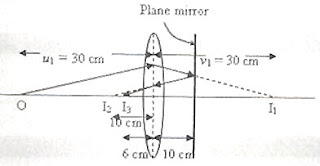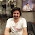## Wednesday, June 23, 2010

### IIT-JEE 2010 Multiple Choice Questions on Optics

Today we will discuss two multiple choice questions on optics, which were included in the IIT-JEE 2010 question paper. The first question is single correct choice type mcq where as the second one is multiple correct choice type mcq.

(1) A biconvex lens of focal length 15 cm is in front of a plane mirror. The distance between the lens and the mirror is 10 cm. A small object is kept at a distance of 30 cm from the lens. The final image is

(A) virtual and at a distance of 16 cm from the mirror

(B) real and at distance of 16 cm from the mirror

(C) virtual and at a distance of 20 cm from the mirror

(D) real and at a distance of 20 cm from the mirror

The lens has focal length f = 15 cm and the object O is placed at distance 30 cm which is equal to 2f. Therefore a real image I1 will be formed at distance 2f (= 30 cm) on the other side of the lens, if the mirror is absent.

Since the image I1 is at 20 cm from the plane mirror, a real image I2 would be formed (by the reflection of the rays at the plane mirror) at distance 20 cm from the mirror. But the returning rays are further refracted by the lens and the final image is formed at I3. The image I2 serves as the object for the lens for the formation of the final image I3.As is clear from the figure, the object distance u3 for the formation of the final image is 10 cm. Therefore we have

1/v3 – 1/10 = 1/15

[We have used the law of distances 1/v – 1/u = 1/f, in accordance with the Cartesian sign convention]

Therefore, 1/v3 = 1/15 + 1/10

This gives v3 = 6 cm.

The image is real and its distance from the plane mirror is (6+10) cm = 16 cm [Option (b)].

(2) A ray OP of monochromatic light is incident on the face AB of prism ABCD near the vertex B at an incident angle of 60º (see figure). If the refractive index of the material of the prism is 3 , which of the following is (are) correct ?

(A) The ray gets totally internally reflected at face CD

(B) The ray comes out through face AD

(C) The angle between the incident ray and the emergent ray is 90º

(D) The angle between the incident ray and the emergent ray is 120º

When the angle of incidence (at P) is 60º, the angle of refraction r is 30º since the refractive index n of the material of the prism is given by

n = sin i/sin r

Or, √3 = sin 60º/sin r so that sin r = (sin 60º)/√3 = ½ from which r = 30ºFrom the quadrilateral PABQ (fig.) it follows that angle PQB = 45º. Therefore the angle of incidence of the ray at the point Q is 45º. But this is greater than the critical angle for the interface.

[If θc is the critical angle for the interface, we have 1/sin θc = n =3 so that sin θc = 1/√3. Since sin 45º = 1/√2 it follows that 45º > θc]

The ray incident at Q is therefore totally reflected and it is incident at an angle of 30º at the point R. The angle of refraction at R is 60º and hence the angle between the incident ray and the emergent ray is 90º.

Options A, B and C are therefore correct.

[If you know the action of the prism commonly used in the constant deviation spectrograph, you will be able to answer this question immediately].

1.2.